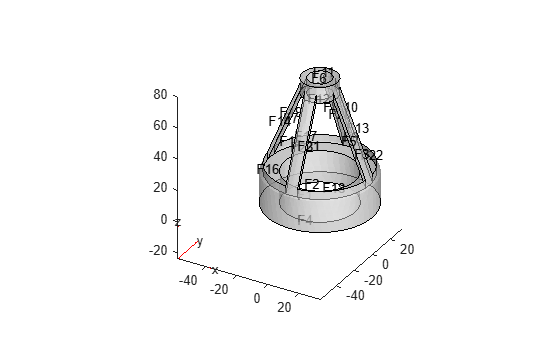## Geometry from Triangulated Mesh

### 3-D Geometry from Finite Element Mesh

This example shows how to create an `fegeometry` object and a `DiscreteGeometry` object from a 3-D mesh.

The `tetmesh` file, which is included in Partial Differential Equation Toolbox™, contains a 3-D tetrahedral mesh. Load the data into your workspace.

`load tetmesh`

The imported variable `tet` contains a connectivity list, and the variable `X` contains a matrix of points. Using these variables, create the triangulation representation.

`TR = triangulation(tet,X)`
```TR = triangulation with properties: Points: [1456x3 double] ConnectivityList: [4969x4 double] ```

Create an `fegeometry` object from the `triangulation` object.

`gm = fegeometry(TR)`
```gm = fegeometry with properties: NumFaces: 23 NumEdges: 50 NumVertices: 30 NumCells: 1 Vertices: [30x3 double] Mesh: [1x1 FEMesh] ```

The geometry contains the imported linear mesh.

`gm.Mesh`
```ans = FEMesh with properties: Nodes: [3x1456 double] Elements: [4x4969 double] MaxElementSize: 8.2971 MinElementSize: 1.9044 MeshGradation: [] GeometricOrder: 'linear' ```

To create a more accurate quadratic mesh, use `generateMesh`.

```gm = generateMesh(gm); gm.Mesh```
```ans = FEMesh with properties: Nodes: [3x9338 double] Elements: [10x4708 double] MaxElementSize: 4.9820 MinElementSize: 2.4910 MeshGradation: 1.5000 GeometricOrder: 'quadratic' ```

Plot the geometry with the face labels.

`pdegplot(gm,FaceLabels="on",FaceAlpha=0.5);`Alternatively, you can create a geometry as a `DiscreteGeometry` object. First, create data matrices of the appropriate sizes.

```nodes = X'; elements = tet';```

Then, create a PDE model and use `geometryFromMesh` to create a geometry from the mesh.

```model = createpde; gm = geometryFromMesh(model,nodes,elements)```
```gm = DiscreteGeometry with properties: NumCells: 1 NumFaces: 23 NumEdges: 50 NumVertices: 30 Vertices: [30x3 double] ```

The model contains the imported linear mesh.

`model.Mesh`
```ans = FEMesh with properties: Nodes: [3x1456 double] Elements: [4x4969 double] MaxElementSize: 8.2971 MinElementSize: 1.9044 MeshGradation: [] GeometricOrder: 'linear' ```

To create a more accurate quadratic mesh, use `generateMesh`.

`generateMesh(model)`
```ans = FEMesh with properties: Nodes: [3x9338 double] Elements: [10x4708 double] MaxElementSize: 4.9820 MinElementSize: 2.4910 MeshGradation: 1.5000 GeometricOrder: 'quadratic' ```

### 2-D Multidomain Geometry

This example shows how to create a 2-D multidomain geometry from a planar mesh.

The `MultidomainMesh2D` file, which is included in Partial Differential Equation Toolbox™, contains a 2-D mesh. Load information about nodes, elements, and element-to-domain correspondence into your workspace.

`load MultidomainMesh2D`

Create a PDE model.

`model = createpde;`

Import the mesh into the model.

`geometryFromMesh(model,nodes,elements,ElementIdToRegionId);`

Plot the geometry with the face labels.

`pdegplot(model,FaceLabels="on")`